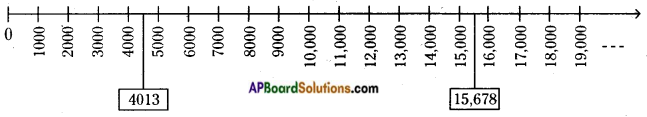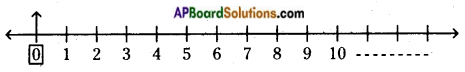AP State Syllabus AP Board 6th Class Maths Solutions Chapter 2 Whole Numbers Ex 2.1 Textbook Questions and Answers.

## AP State Syllabus 6th Class Maths Solutions 2nd Lesson Whole Numbers Exercise 2.1

Question 1.
How many whole numbers are there in between 27 and 46?
Number of whole numbers upto 27 is 28 (from zero to 27)
Number of whole numbers upto 45 is 46 (excluding 46)
Number of whole numbers between 27 and 46 = 46 – 28 = 18
They are 28, 29, 30, 31, 32, 33, 34, 35, 36, 37, 38, 39, 40, 41, 42, 43, 44, 45.Question 2.
Find the following using number line.
i) 6 + 7 + 7
ii) 18 – 9
iii) 5 × 3
i) 6 + 7 + 7
Draw the number line starting from zero (0).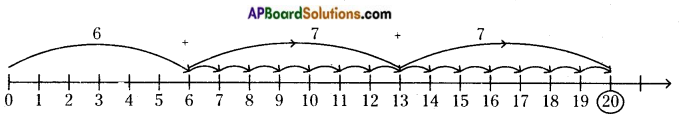Starting from 6, we make 7 jumps to the right of 6 on the number line. Then we reach 13. Again make 7 jumps to the right of 13. Then we reach 20.
So, 6 + 7 + 7 = 20

ii) 18 – 9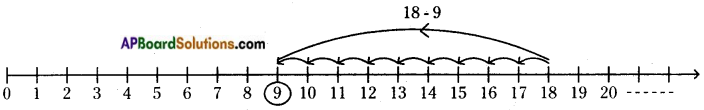Draw the number line starting from zero (0).
Start from 18. We make 9 jumps to the left of 18 on the number line.
Then we reach 9.
So, 18 – 9 = 9iii) 5 × 3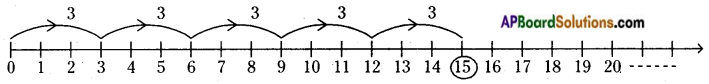Draw the number line starting from zero (0).
Start from 0 and make 3 jumps to the right of the zero on the number line.
Now, treat 3 jumps as one step.
So, to make 5 steps (i.e., 3, 6, 9, 12 and 15) move on the right side, we read 15 on the number line.
So, 5 × 3 = 15

Question 3.
In each pair, state which whole number on the number line is on the right of the other number.
i) 895, 239
As 239 < 895, we conclude that 895 is on the RHS of 239.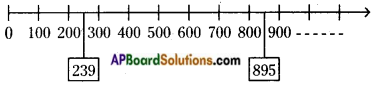895 is right of 239 on the number line.

ii) 1001, 10001
As 1001 < 10001, we conclude that 10001 is on the RHS of 1001.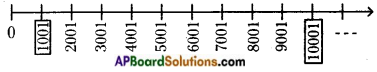10001 is right of 1001 on the number line.

iii) 15678, 4013